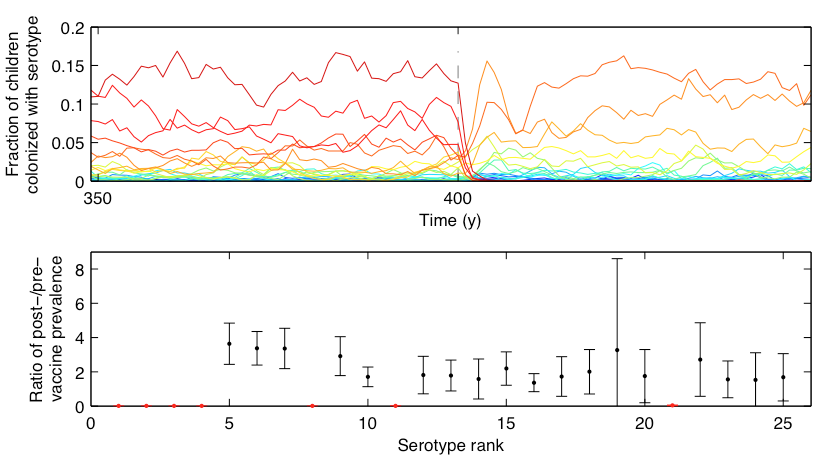## Fitting models to time series

### A general approach to model fitting

Pick a model, including parameter values

Evaluate how likely the observed data are, given the model

Tweak the model to make the observations more likely

Is this model superior to other models?

### Maximum likelihood (ML) inference

In ML, you have some set of data $D$ and a model for generating this data. This model has parameters $\theta$. The probability of observing data is $\mathrm{Pr}(D \, | \, \theta)$. The best parameter point estimate $\hat{\theta}$ is simply the value that maximizes $\mathrm{Pr}(D \, | \, \theta)$.

### Maximum likelihood (ML) inference

For example, if we have data $D$ from a Bernoulli observation model representing $k$ successes in $n$ trials, then the probability of observing $k$ and $n$ given coin flip probability parameter $p$ is simply $$\mathrm{Pr}(k,n \, | \, p) = p^k \, (1-p)^{n-k}.$$

### Maximum likelihood (ML) inference

For the Bernoulli model $\mathrm{Pr}(k,n \, | \, p) = p^k \, (1-p)^{n-k}$, we have $\hat{p} = k/n$. For example, with $k=8$ and $n=10$, $\hat{p}=0.8$ the likelihood curve follows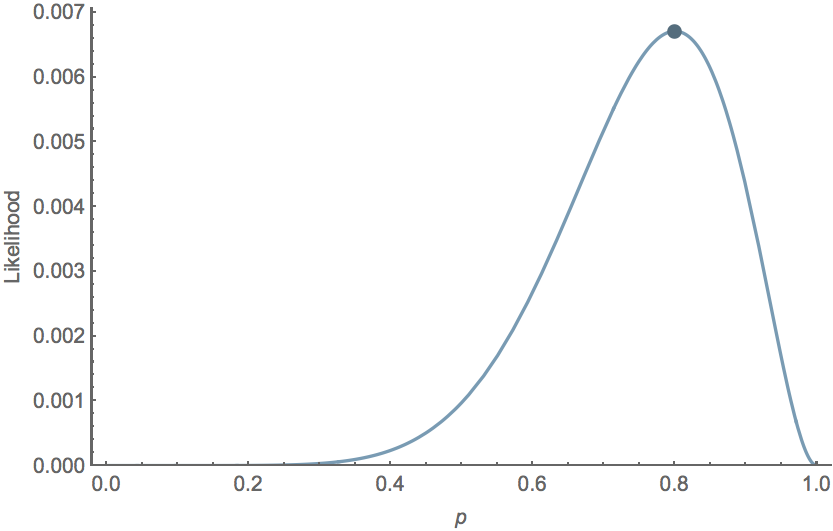### Likelihood in timeseries models

Observed trajectory $D=(t_0,...,t_n)$ depends on unknown parameter(s) $\theta$

Probability density function of $D$ is $f_{\theta}$

$$L_D(\theta)=f_{\theta}(D)$$

Problem: These data aren't independent.

### Likelihood from non-independent data

Solution: Factor the joint density into conditional and marginal

e.g., $f(y_3,y_2,y_1)=f(y_3 \, | \, y_2,y_1) \cdot f(y_2,y_1)$

$$f(y_3,y_2,y_1)=f(y_3 \, | \, y_2,y_1) \cdot f(y_2 \, | \, y_1) \cdot f(y_1)$$

$$L(\theta)=\prod_{t=2}^{T}f(y_t|l_{t-1})\cdot f(y_1)$$

where $l_{t-1}$ is information through $t-1$ (i.e., $y_{t-1},...,y_1$), and $T$ is the time series length

### What's $D$?

Case counts at different times

Sequences

Titers

or some composite of observations

### Maximizing the likelihood

means maximizing the log-likelihood

or minimizing the negative log-likelihood

### Finding the maximum likelihood

Can be analytically tractable

For our models, it's not

### General approaches to likelihood maximization

Brute force

Derivative-based methods

Simplex

Simulated annealing

Sequential Monte Carlo

Many others... but few tried and true

### Inference for time series

POMP (partially observed Markov Process)

pMCMC (particle Markov chain Monte Carlo)

TSIR, if conditions met

### Interacting dengue serotypes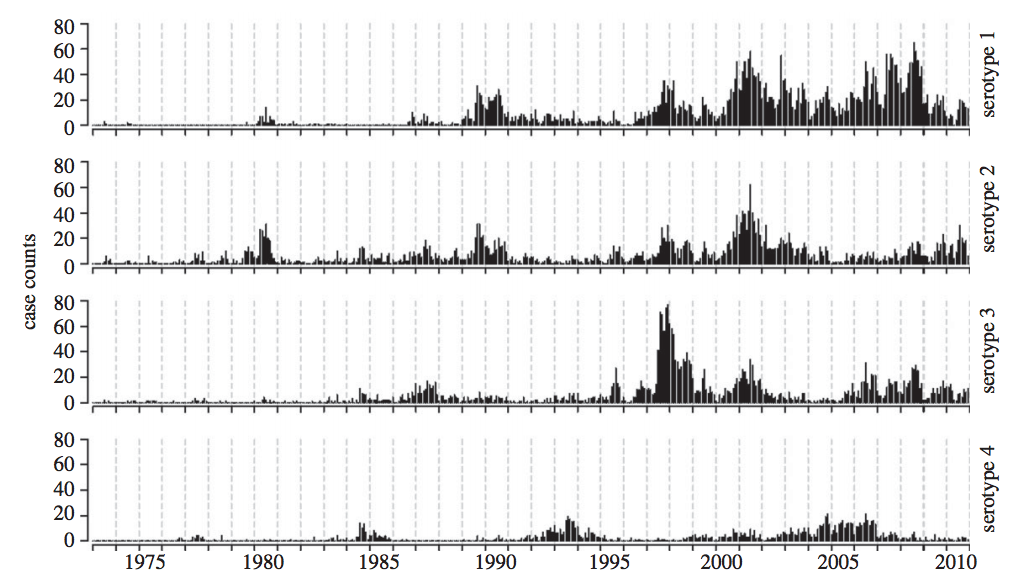### Likelihood profiles

Hold parameter(s) constant, fit the rest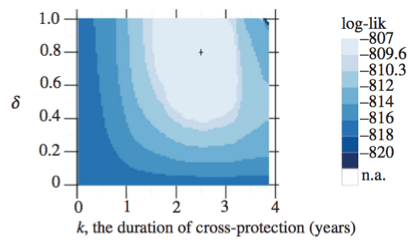### Bayesian inference

Generally, it's difficult to make probability statements using frequentist statistics. You cannot directly say that model 1 is twice as likely as model 2. People misuse p values in this sort of fashion all the time.

### Bayes' rule

Bayes' rule forms the basis of Bayesian inference, it states: $$\mathrm{Pr}(A \, | \, B) = \cfrac{ \mathrm{Pr}(B \, | \, A) \, \mathrm{Pr}(A) }{ \mathrm{Pr}(B) }$$

### Bayesian inference

Bayesian inference applies Bayes' rule in a likelihood context, so that $$\mathrm{Pr}(\theta \, | \, D) = \cfrac{ \mathrm{Pr}(D \, | \, \theta) \, \mathrm{Pr}(\theta) }{ \mathrm{Pr}(D) },$$ where $D$ is data and $\theta$ are parameters. $\mathrm{Pr}(D)$ is constant with respect to $\theta$, so that $\mathrm{Pr}(\theta \, | \, D) \propto \mathrm{Pr}(D \, | \, \theta) \, \mathrm{Pr}(\theta)$. This relationship is often referred to as $\mathrm{posterior} \propto \mathrm{likelihood} \times \mathrm{prior}$.

### Bayesian inference for Bernoulli model

Following our previous Bernoulli example, we've observed $k$ successes in $n$ trials, and so the likelihood $\mathrm{Pr}(k,n \, | \, p) = p^k \, (1-p)^{n-k}$. We'll assume a flat prior $\mathrm{Pr}(p) = 1$. In this case, the marginal likelihood follows $$\mathrm{Pr}(k,n) = \int_0^1 \mathrm{Pr}(k,n \, | \, p) \, \mathrm{Pr}(p) \, dp = \cfrac{k! \, (n-k)!}{(n+1)!}.$$ And the full posterior follows $$\mathrm{Pr}(p \, | \, k,n) = \cfrac{(n+1)! \, p^k \, (1-p)^{n-k}}{k! \, (n-k)!}.$$

### Bayesian inference allows for probability statements

If $k=8$ and $n=10$, the mean posterior $\mathrm{E}[p] = 0.75$, while the 95% credible interval extends from $0.482$ to $0.896$, and the posterior distribution follows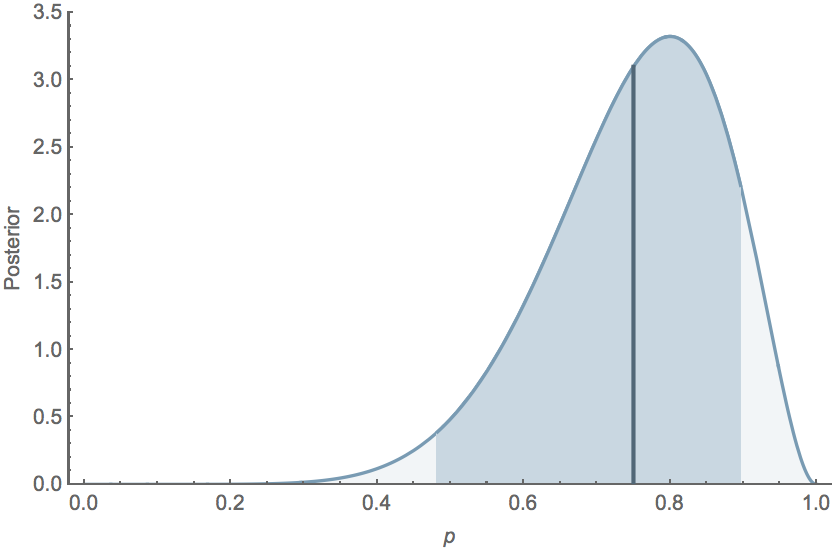### Maximum likelihood (ML) inference

For the Bernoulli model $\mathrm{Pr}(k,n \, | \, p) = p^k \, (1-p)^{n-k}$, we have $\hat{p} = k/n$. For example, with $k=8$ and $n=10$, $\hat{p}=0.8$ the likelihood curve follows### Methods for Bayesian integration

Markov Chain Monte Carlo

Metropolis-Hastings MCMC

Particle MCMC

Hybrid/Hamiltonian Monte Carlo

Many others

### Simulation-based inference

e.g., R-package pomp

Fit models to time series or (new) longitudinal data

### A simple model of HPV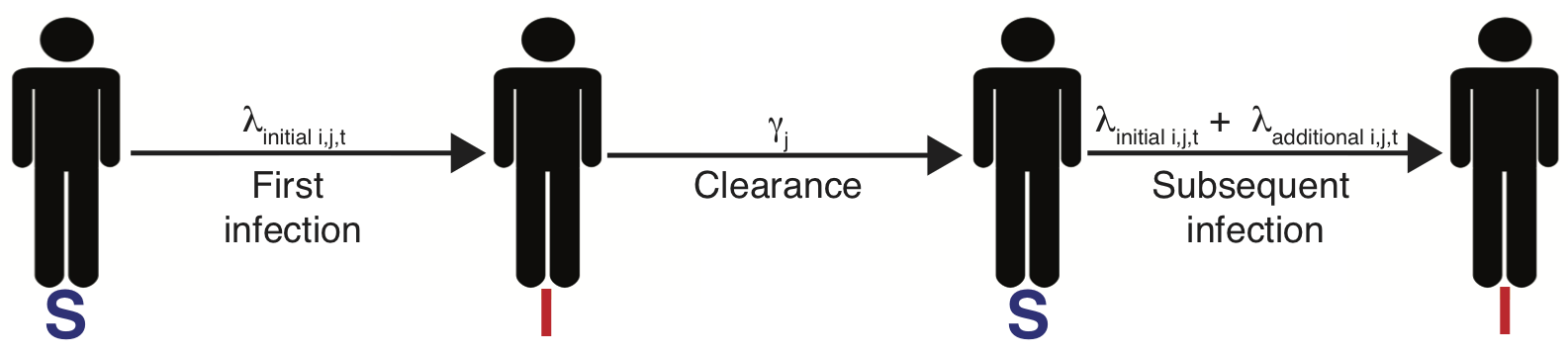$\lambda_{i,j,t}=\lambda_{0_j}f(\overrightarrow{\theta_j} \boldsymbol{X_{it}}) + \text{I(prev. inf.)}d_{jc_i}e^{-w_j(t-t_\mathrm{clr})}$

### Past infection raises risk, even for celibates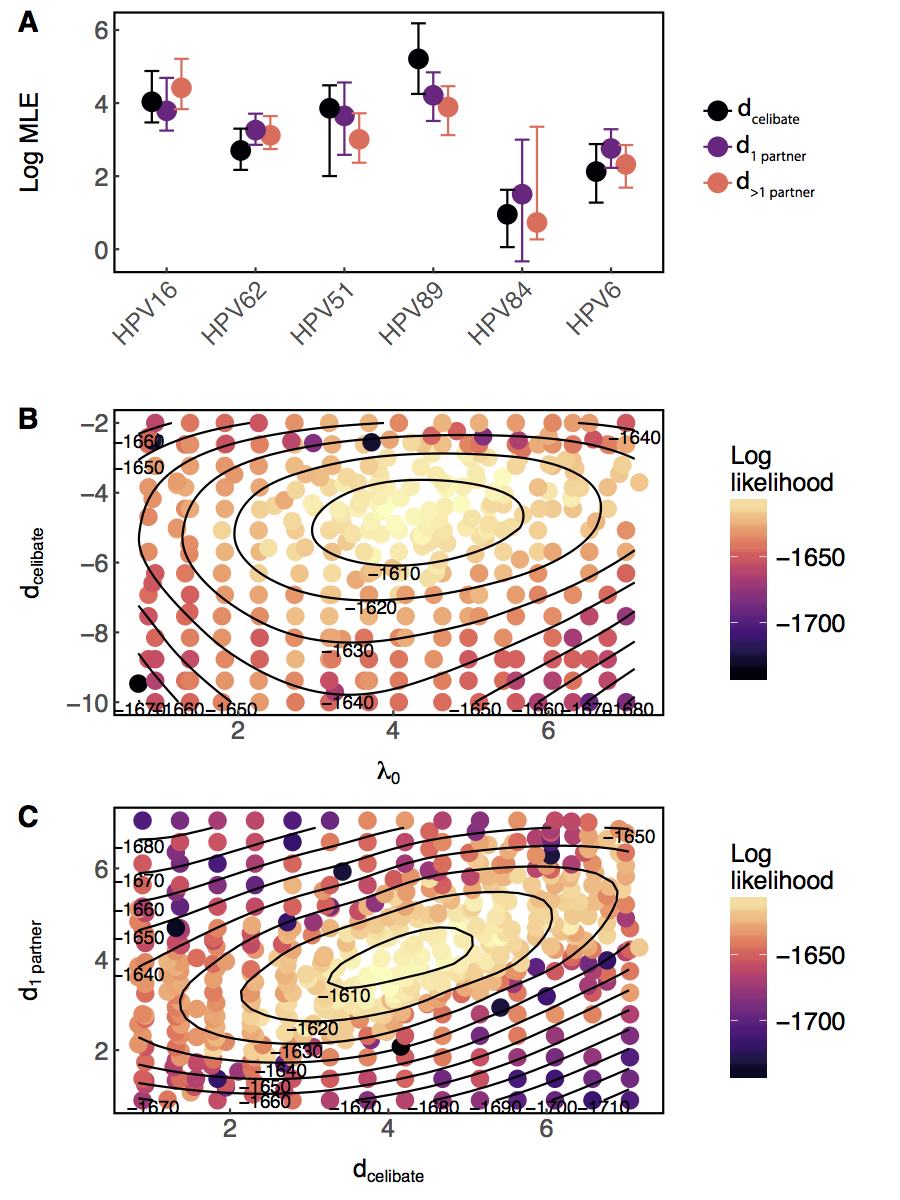### Probes and arbitrary metrics

Approximate Bayesian Computation

Time-series probes

### Phases insufficient to infer interaction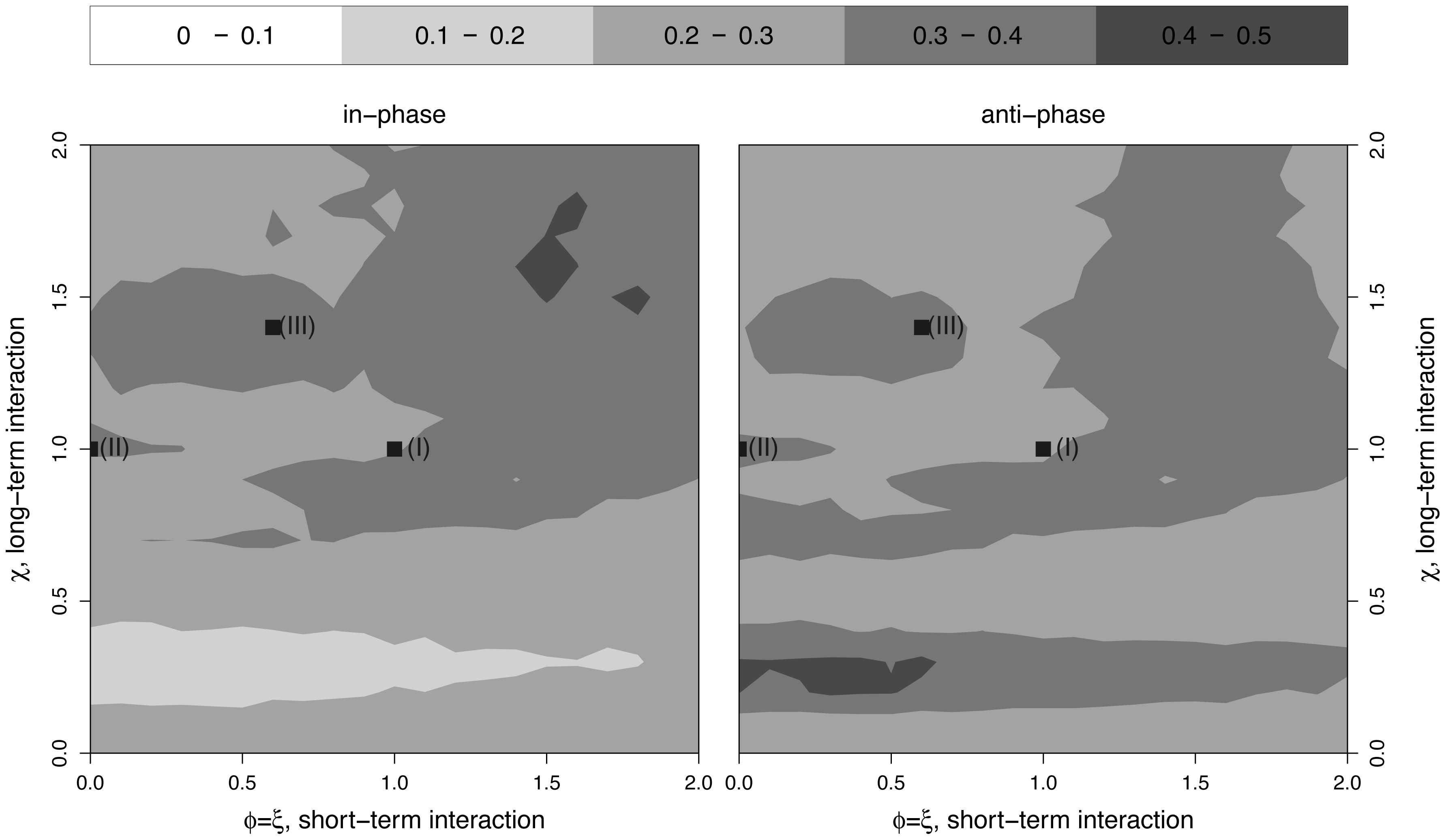### Challenges fitting multistrain models

Many parameters

Multiple minima

Noise, nonstationarity, etc.

### How would we estimate how strongly two strains are competing?

Assume we have time series of cases of each.

### One approach (1/3)

• Assume yesterday's model structure. (Potential modifications: allow coinfections, noise in rates.)
• Create a mapping from latent states (number infected, $X(t)$) to data (cases, $Y(t)$), e.g., $$Y(t) \sim \text{nbinom}(\text{mean} = \rho X(t), \text{size} = \psi )$$
• with $\rho$ representing the reporting rate. (N.B. Potential problems with overcounting here; better to use an accumulator variable.)

### One approach (2/3)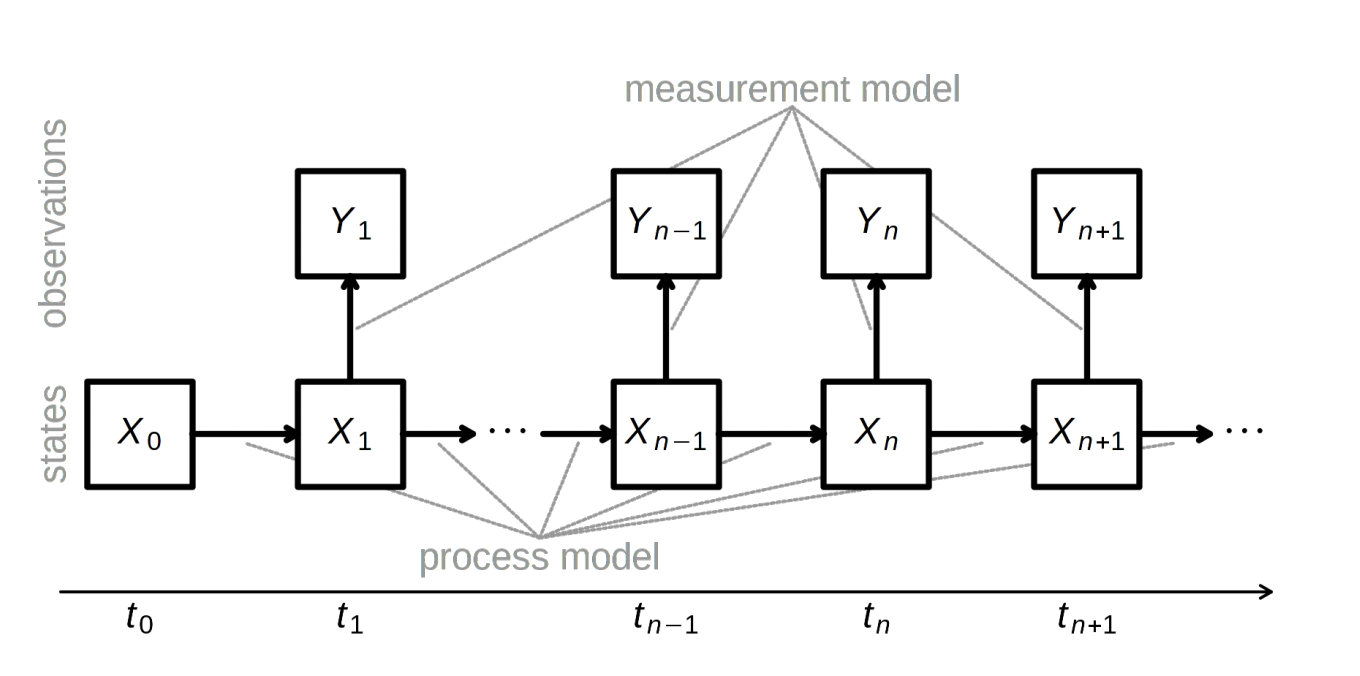### One approach (3/3)

Constrain/fix some parameters, fit (vary) $\alpha$, a, maybe $\rho$, etc., to find the parameter combinations that maximize the likelihood of the data, e.g., multiple iterated filtering using a particle filter (sequential Monte Carlo)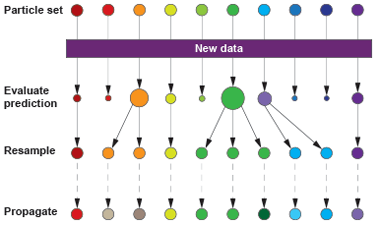### Model "validation"

Confirm convergence

AIC and WAIC

Leave-one-out cross-validation (LOO)

Out-of-sample prediction

Replicate on simulated data

### Gauge the power of your data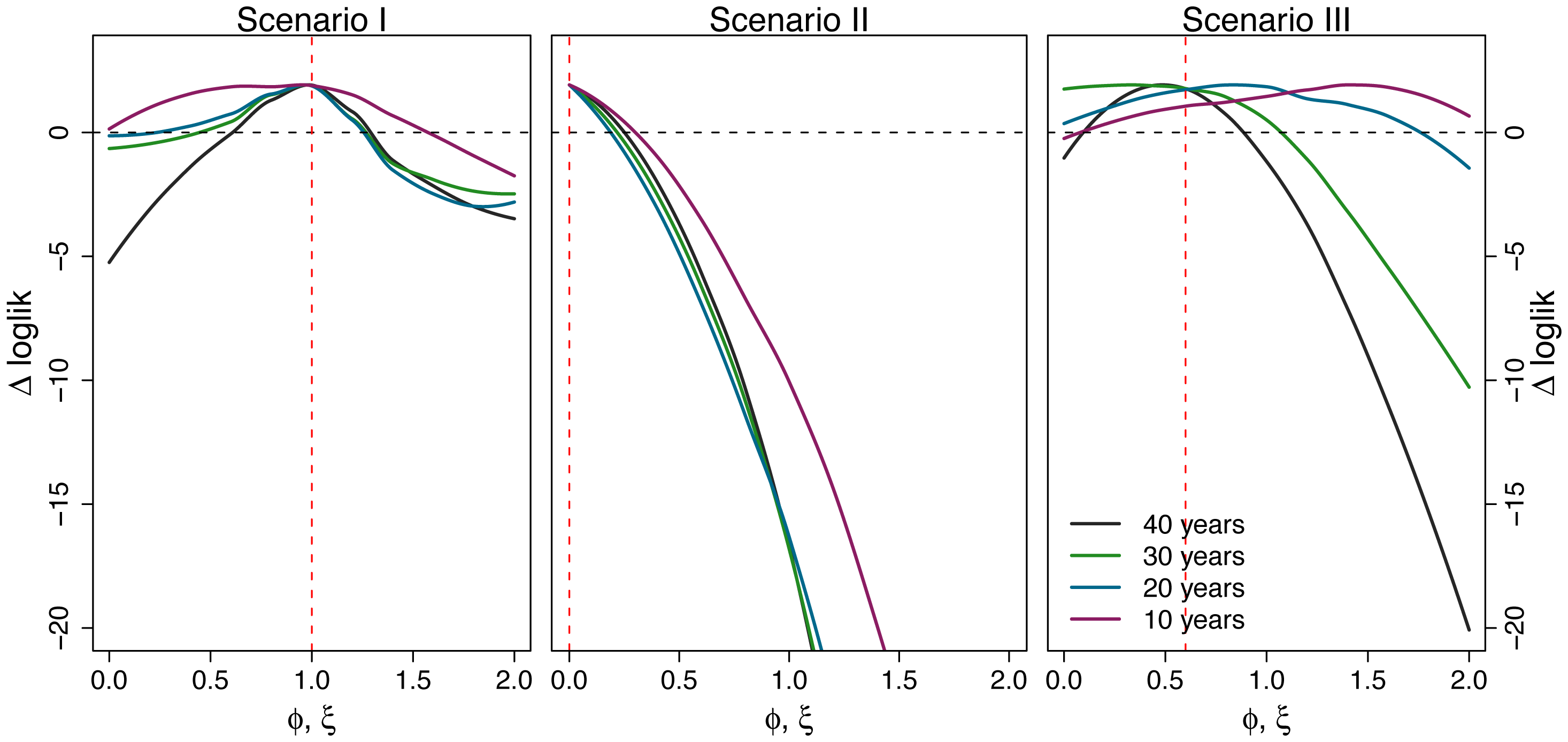## Appendix 1: Inference via state-space reconstruction

### A system with two state variables

$$H'=aH-bHL$$

$$L'=cHL-dL$$

$H$ hares, $L$ lynxes

hare birth rate $a$, predation rate $b$,

consumption rate $c$, death rate $d$

### Solve for H(t), L(t) by numerical integration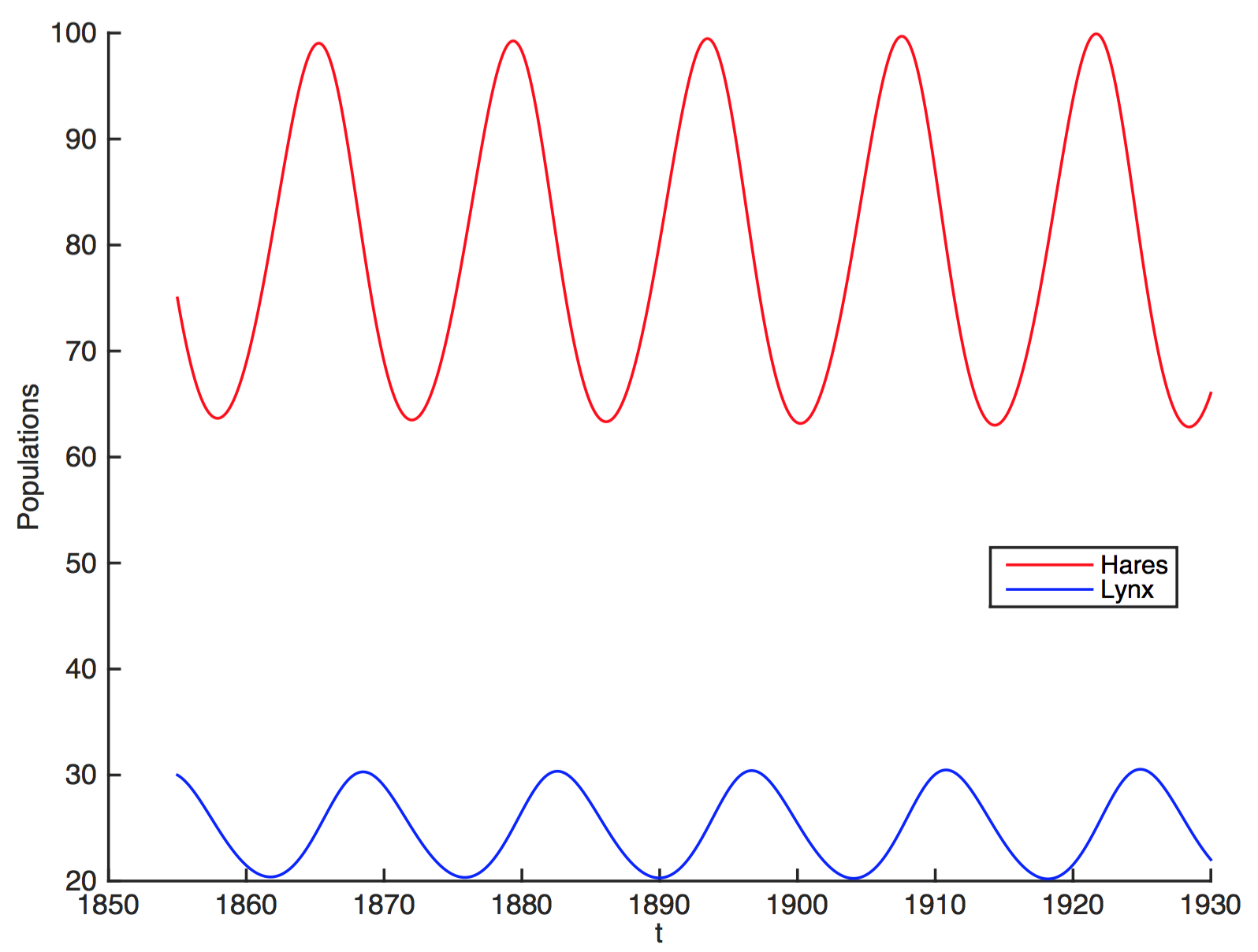### Attractor is a limit cycle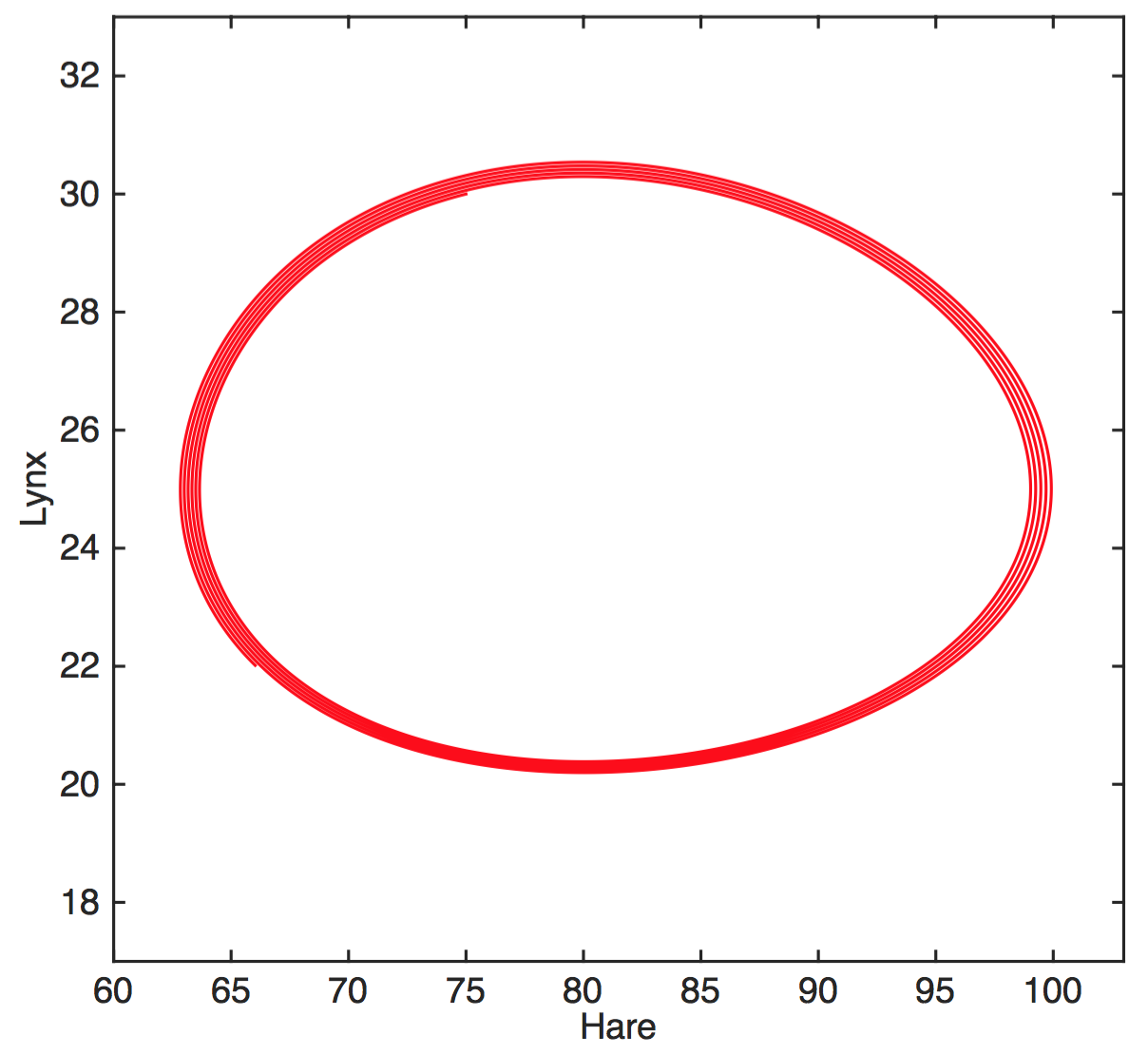### A more complex system

$$x'=\sigma(y-x)$$

$$y'=x(\rho-z)-y$$

$$z'=xy-\beta z$$

### The Lorenz attractor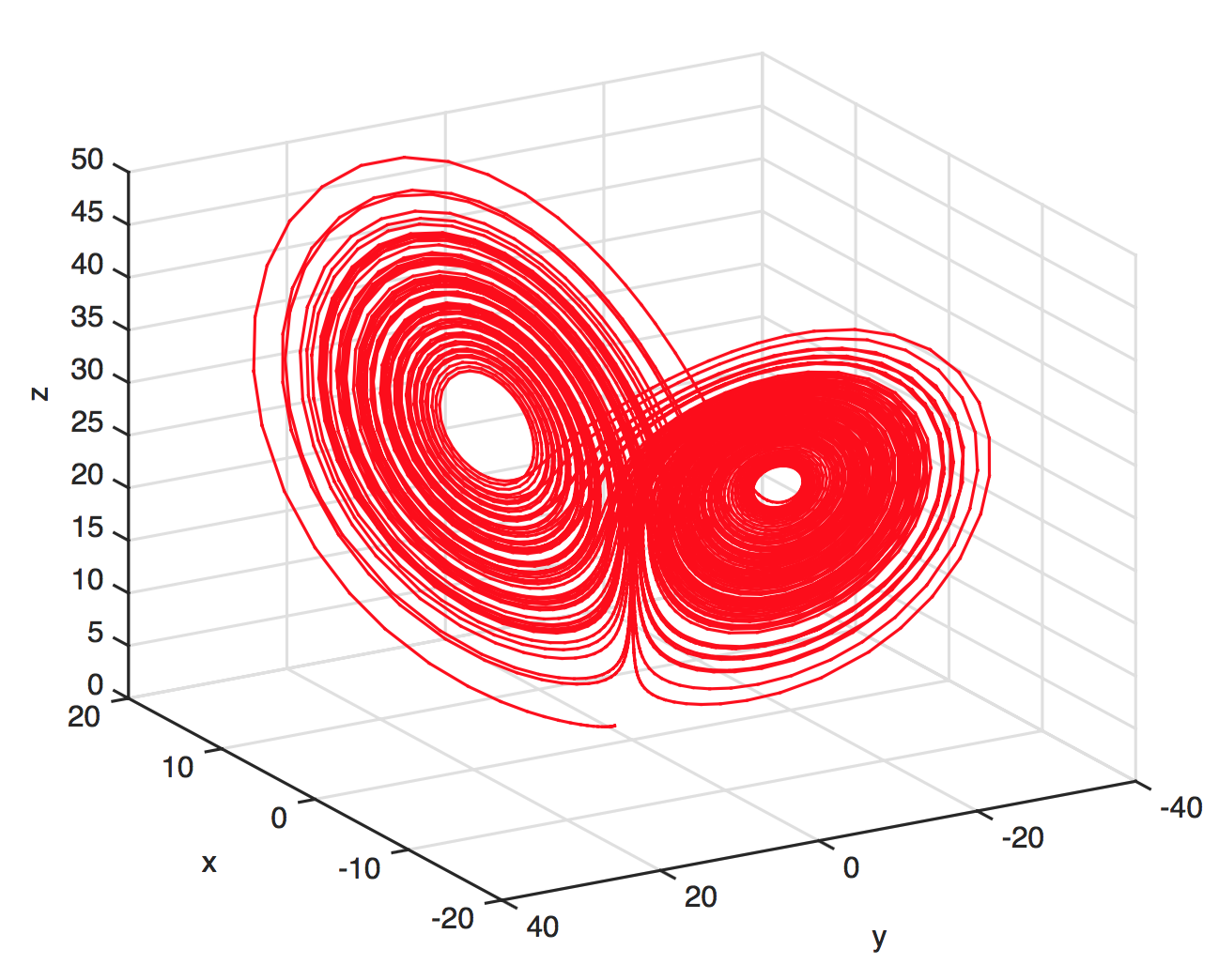### Implications of state-space reconstruction

We can detect underlying structure

We can detect and predict without understanding

New claim: We can infer causal interactions

### Takens' theorem

Very roughly, the system's attractor is diffeomorphic to (can be mapped without loss of information) to the individual attractors of the state variables in some delay-embedding space.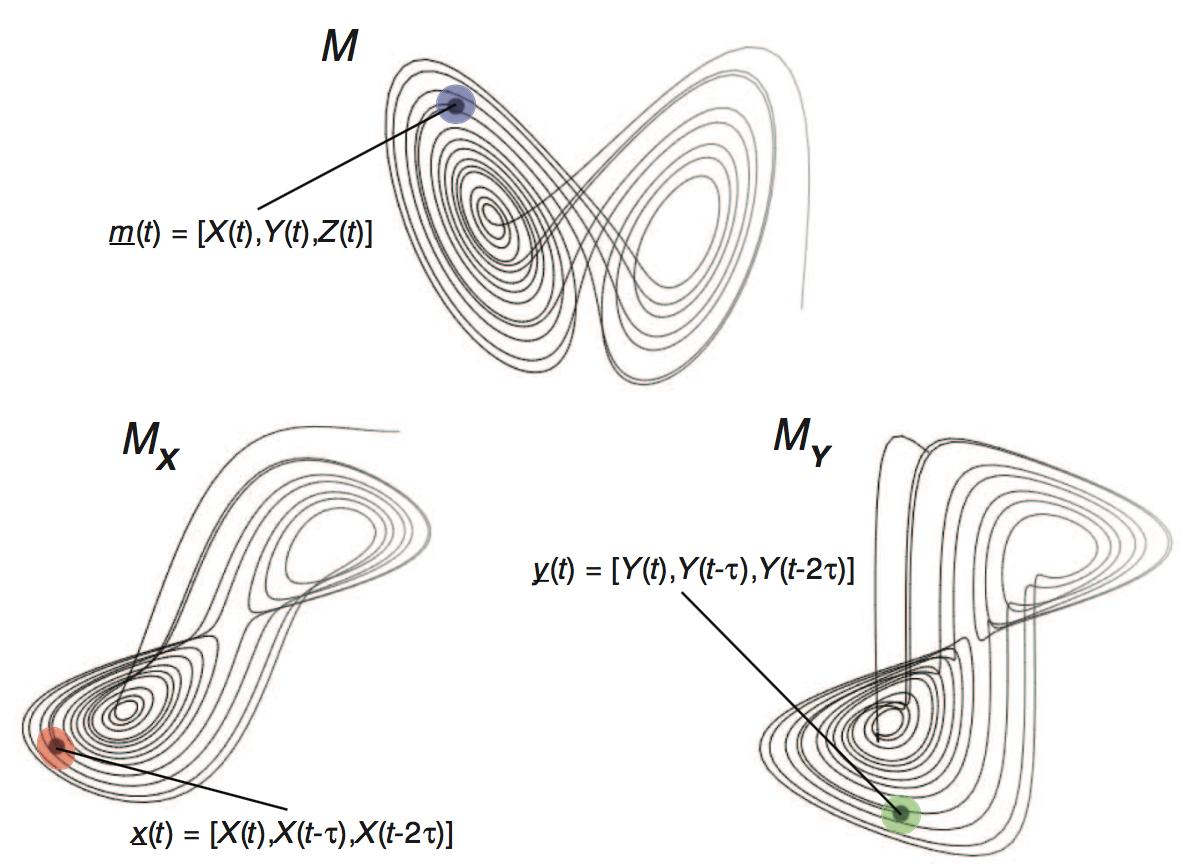### Causal inference from "ecological" data?

Through their shadow manifolds, variables in the same dynamical system can predict each other.

If $X$ drives $Y$, increasing the number of observations of $Y$ should improve predictions of states of $X$.

### Convergent cross-mapping

To infer if $X$ drives $Y$:

• Construct the shadow manifold of $Y$, $\pmb{M}_Y$ (for some $E$, $\tau$). (Each point in $\pmb{M}_Y$ is given by $\vec{y}(t) = \{y_t,y_{t-\tau},y_{t-2\tau},...,y_{t-(E-1)\tau}\}$.)
• For each $X(t)$, identify its analogues $\vec{x}(t)$ and $\vec{y}(t)$.
• Find the $E+1$ nearest neighbors of $\vec{y}(t)$ and weight them by their Euclidean distances to $\vec{y}(t)$.
• To make a prediction $\hat{X}(t)$, multiply these weights by the respective points in $\pmb{M}_X$. Let $\rho$ be the correlation between $\vec{x}(t)$ and $\hat{X}(t)$.
• First make predictions from $\pmb{M}_Y$ constructed with only a few points in the time series, $L_\text{min}$, and then with many, $L_\text{max}$.
• If $\rho$ increases with more information on $\pmb{M}_Y$, $X$ drives $Y$.

### Deterministic toy model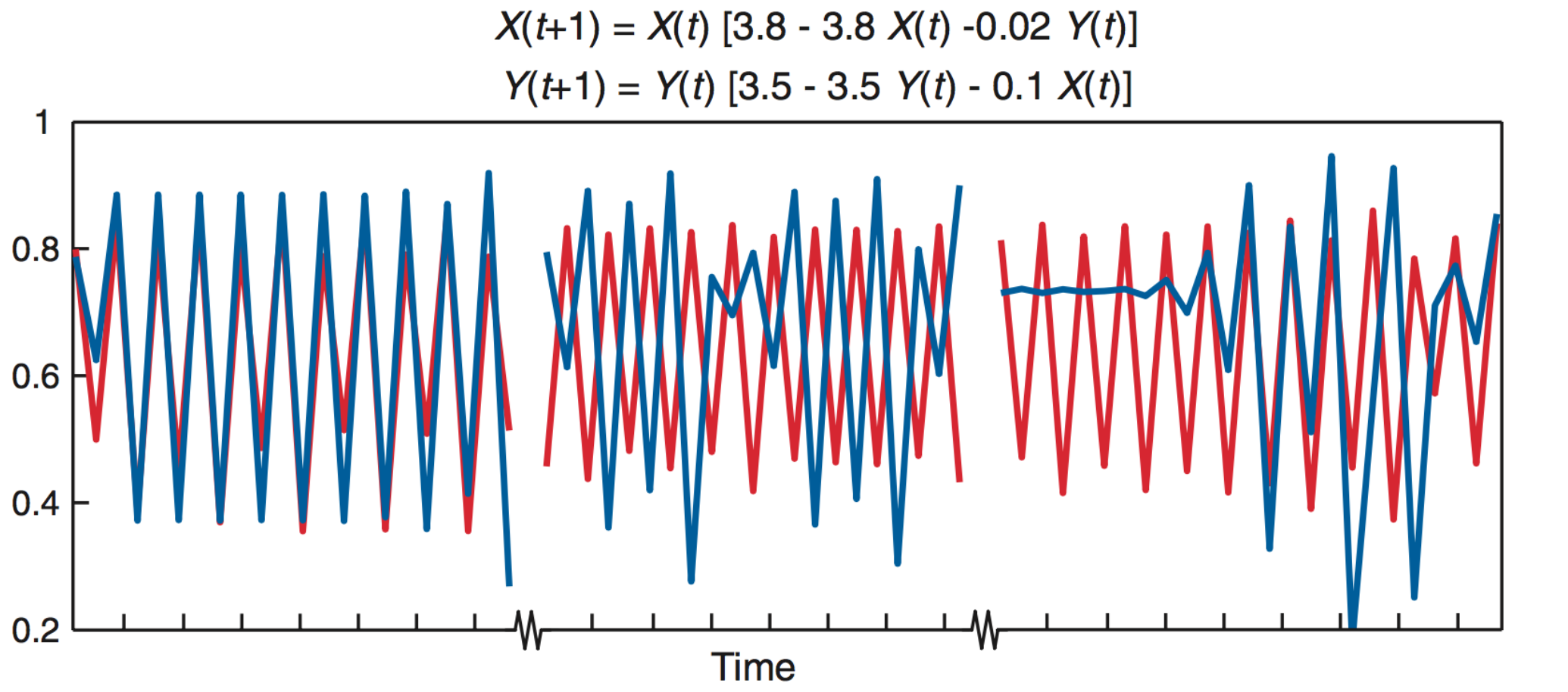### Under determinism, perfect predictability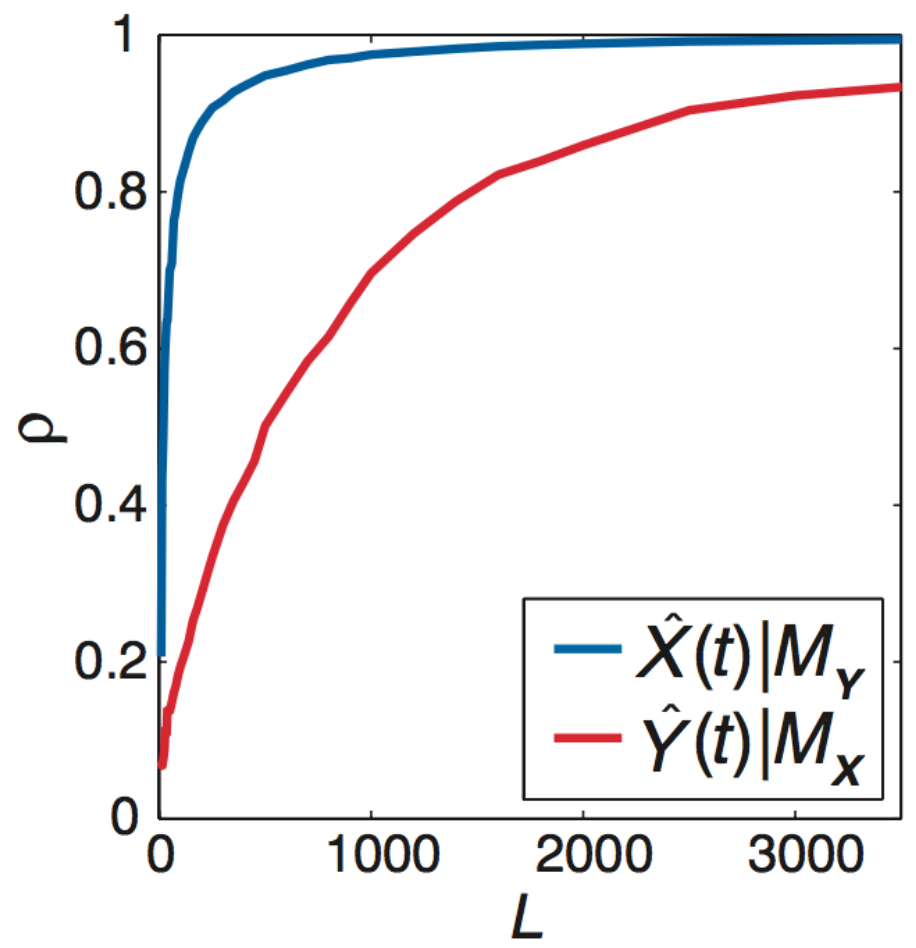### $X$ and $Y$ do not interact but share a driver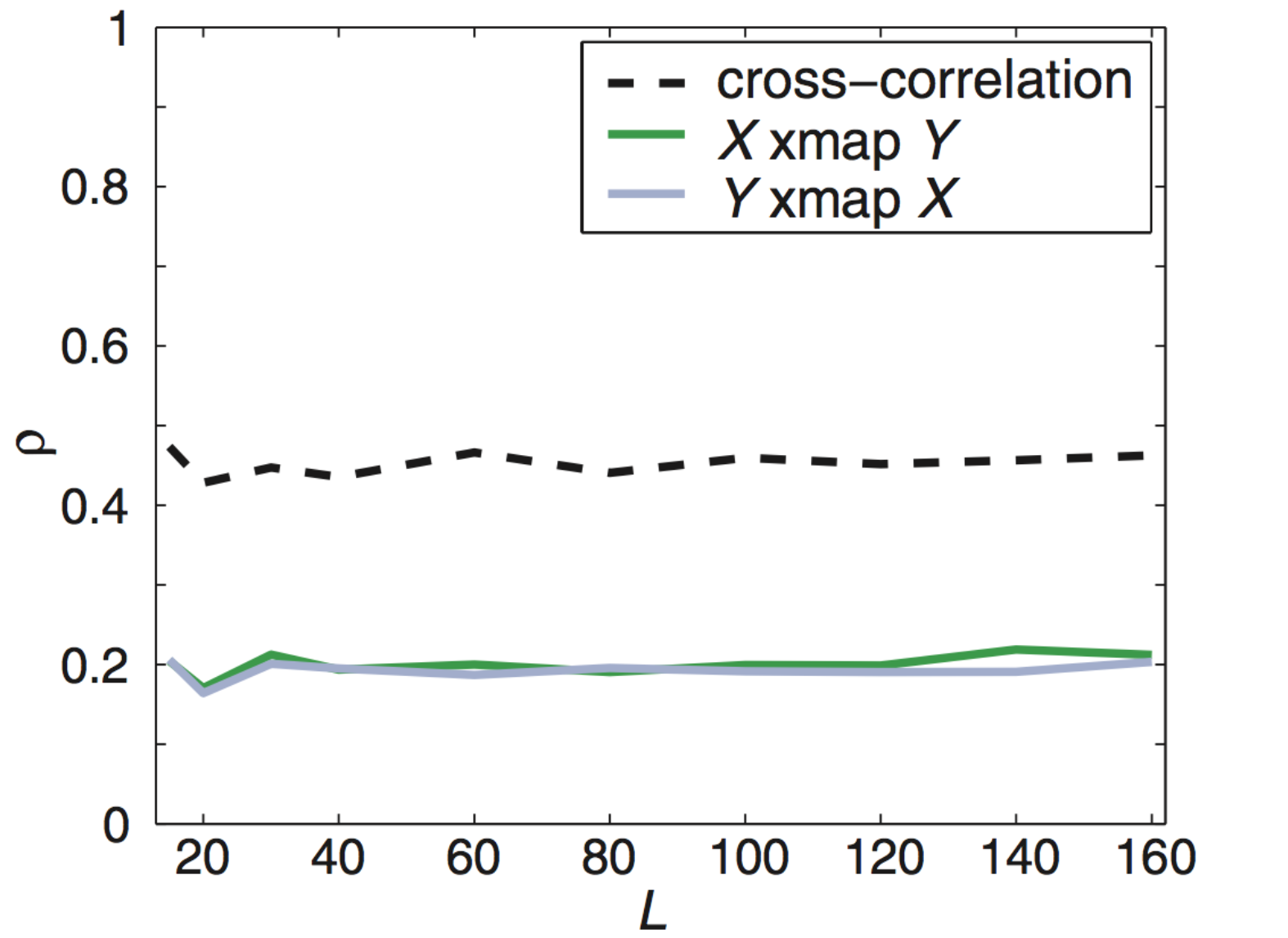### Applied to predator-prey cycles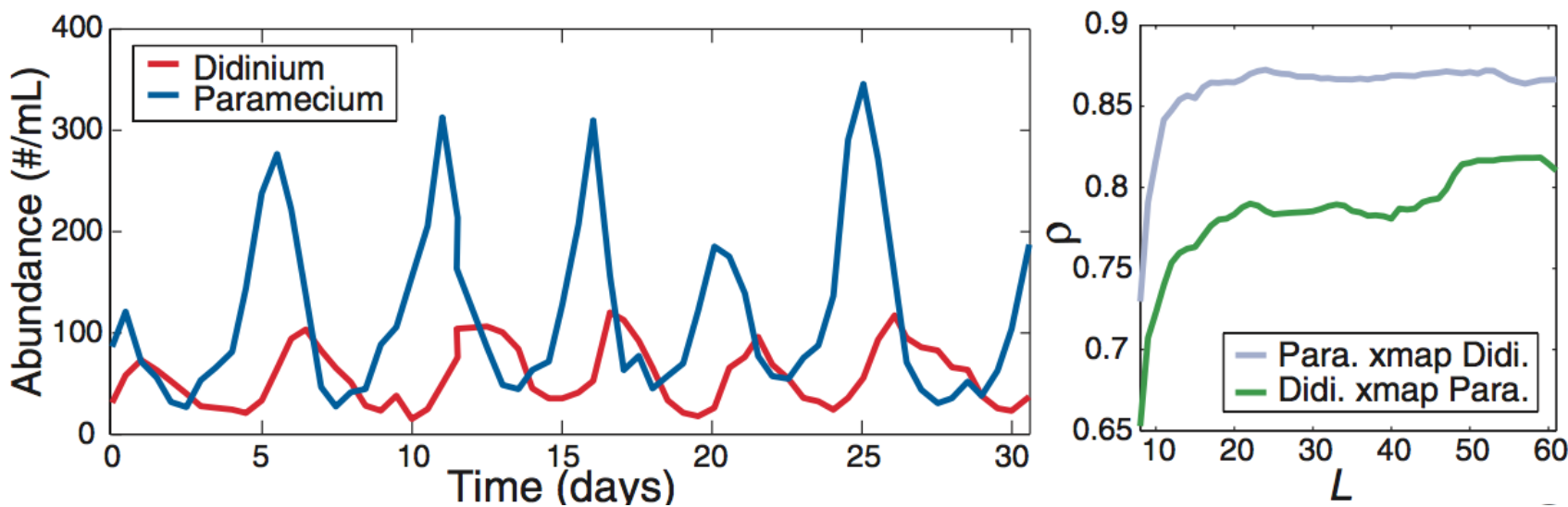### Cross-map lag shows direction### Anchovies, sardines, and SST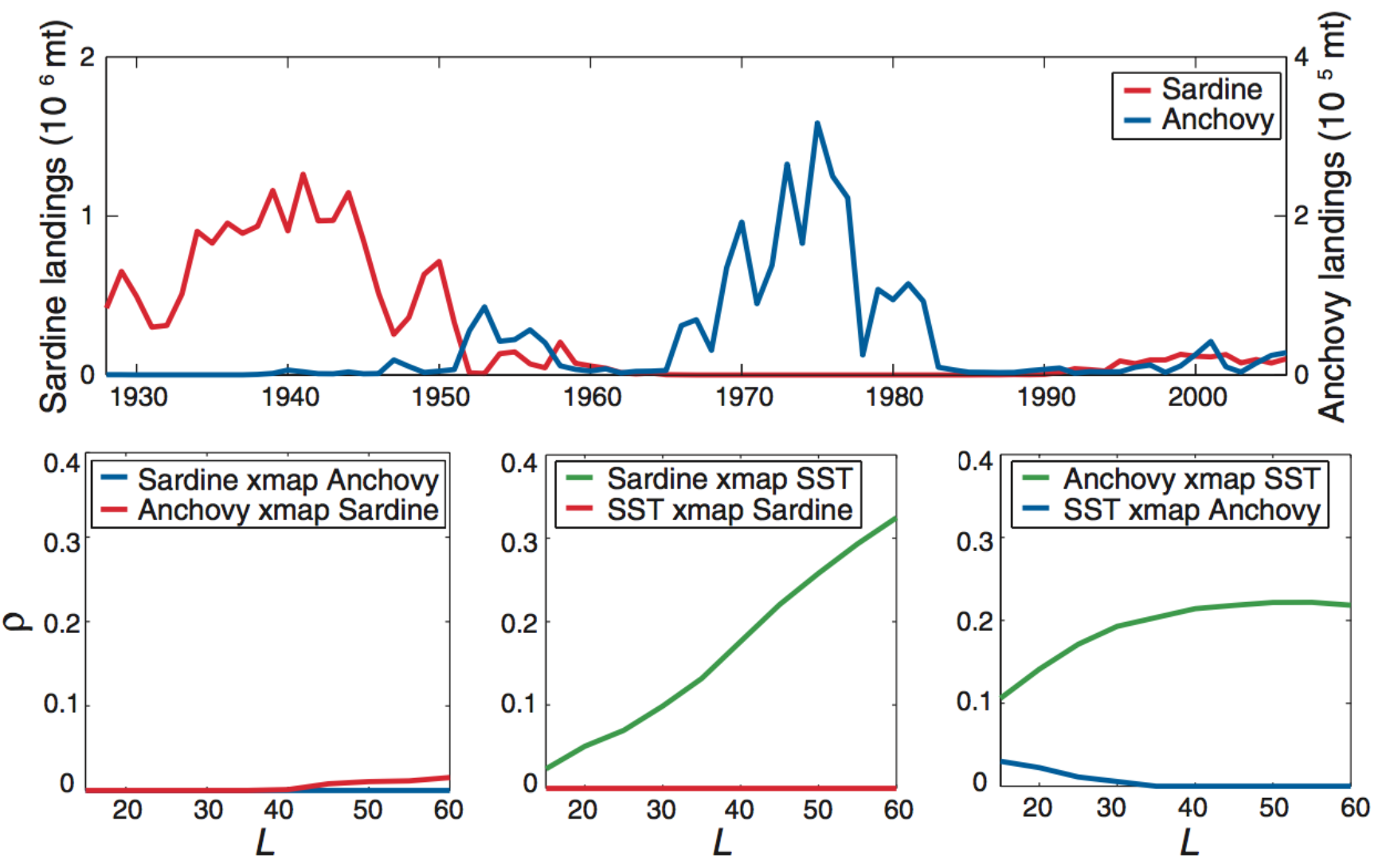### Application to real time series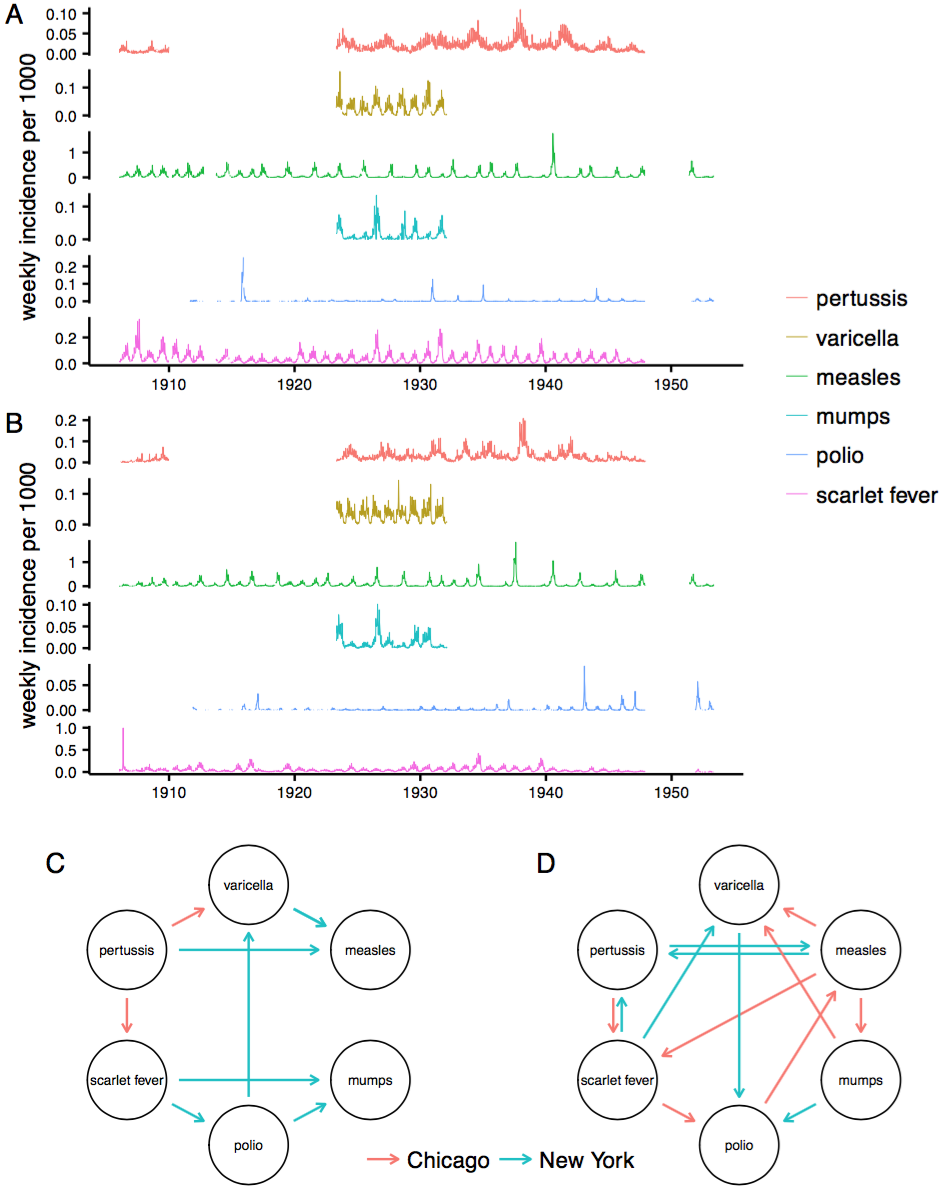### Claim: Absolute humidity drives influenza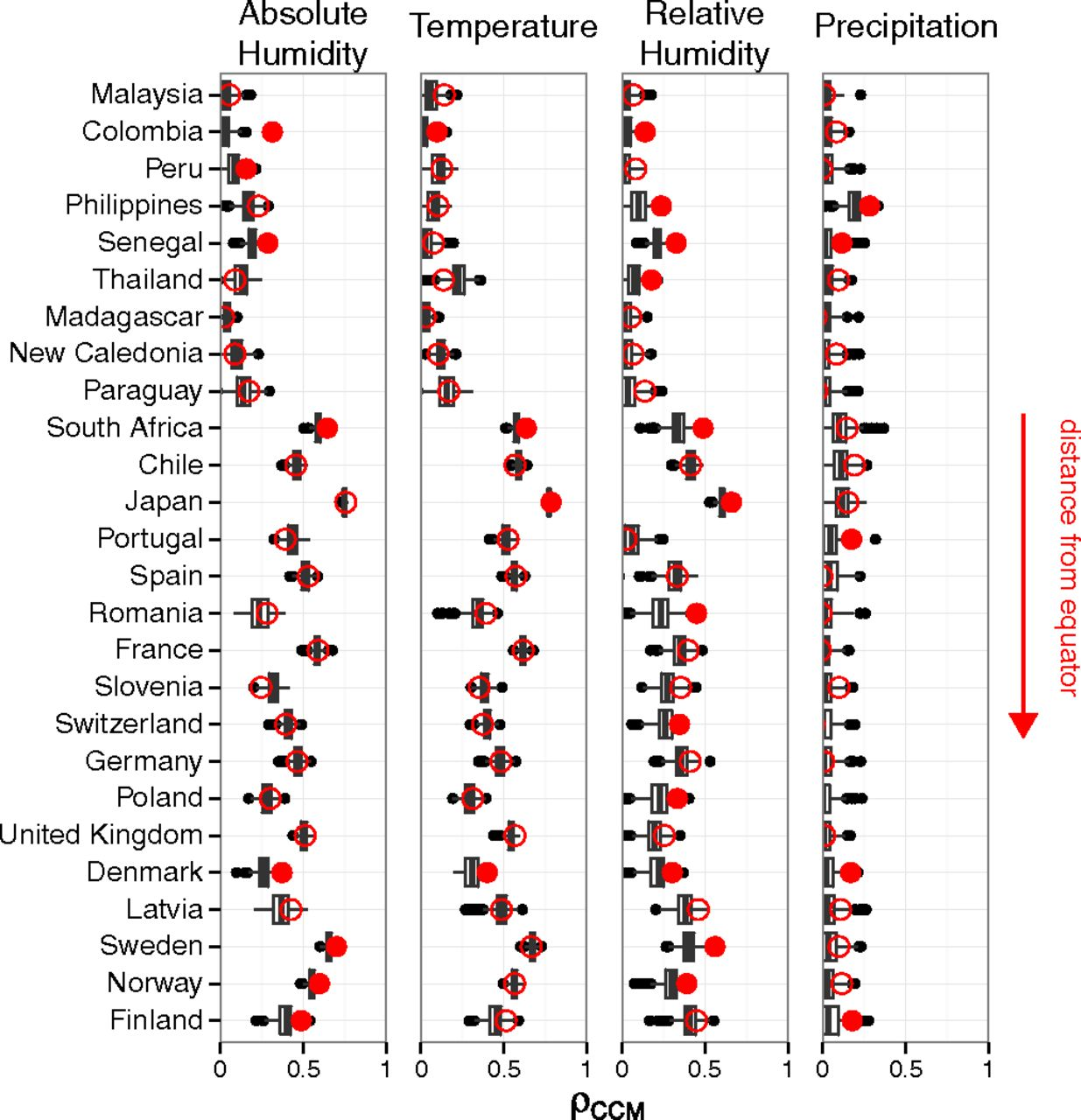### But does flu drive humidity?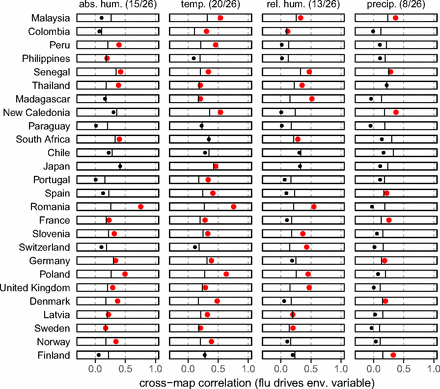## Appendix 2: Example of model validation

### Building confidence in your model

Predict something else

Exploit natural and unnatural disturbances

### Streptococcus pneumoniae

Carried by 20-80% of young children

Transmitted mostly between healthy carriers

>90 serotypes

Some serotypes seem better at everything

### Little evidence for anticapsular immunity### Non-serotype-specific immunity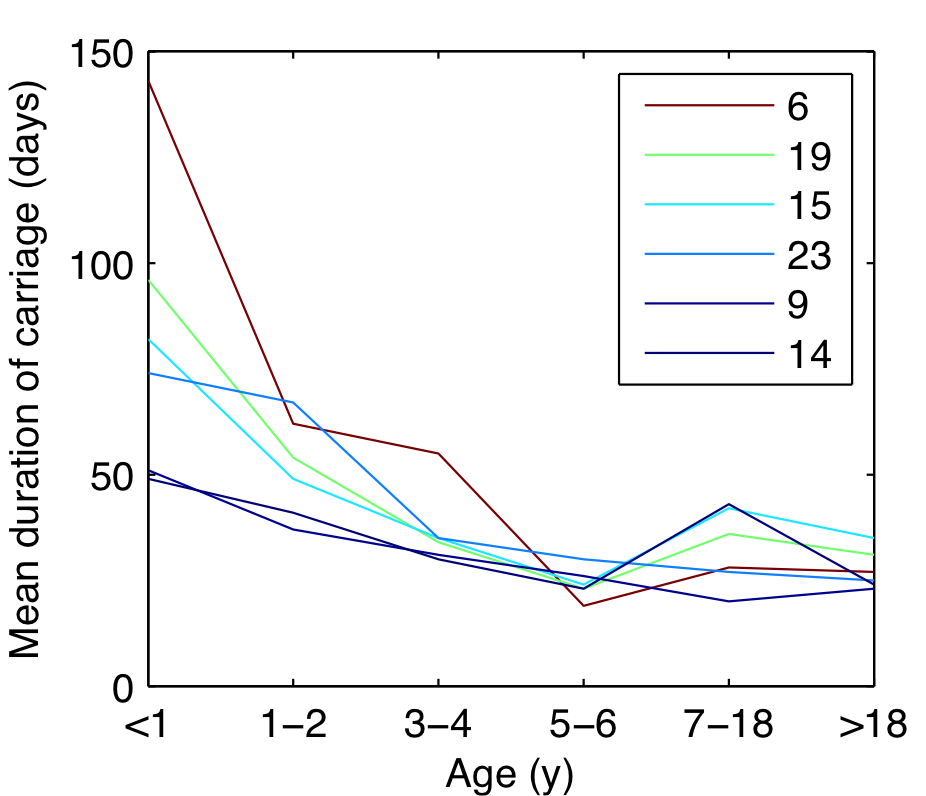### Fitted duration of carriage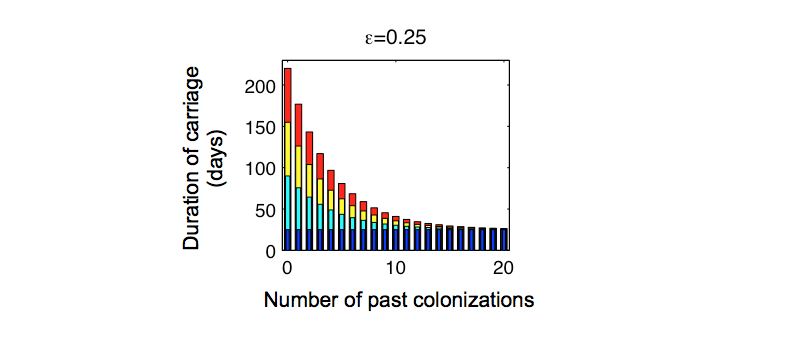### Approach

For each value of serotype-specific immunity, $\sigma$

Fit the transmission rate to obtain 40% prevalence in kids

(Later, sensitivity analysis on fixed parameters)

### Model reproduces diversity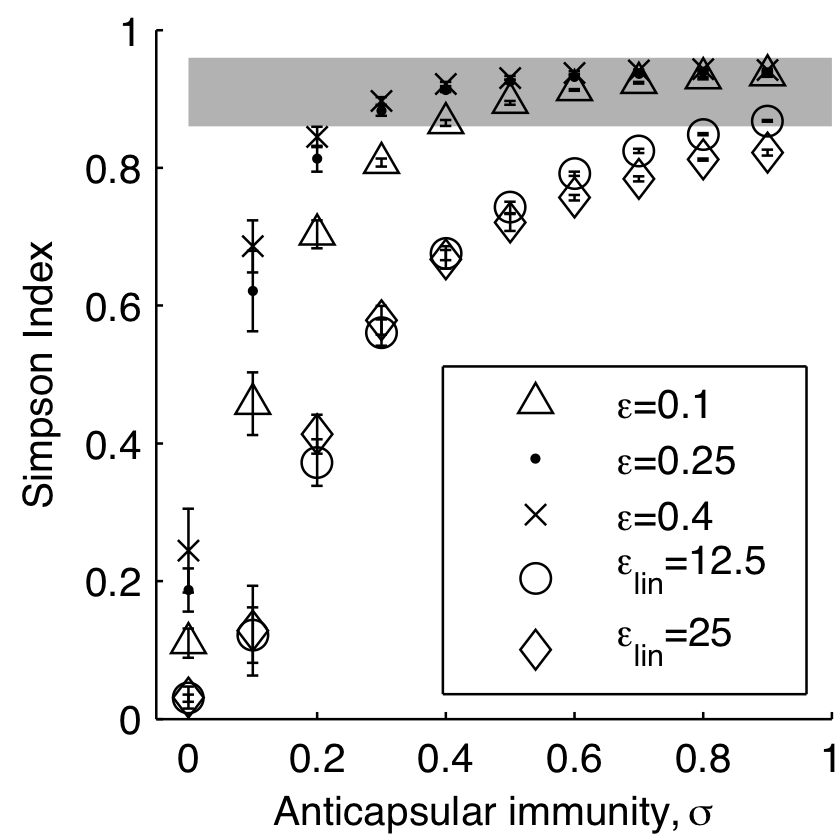### ...including rank-frequency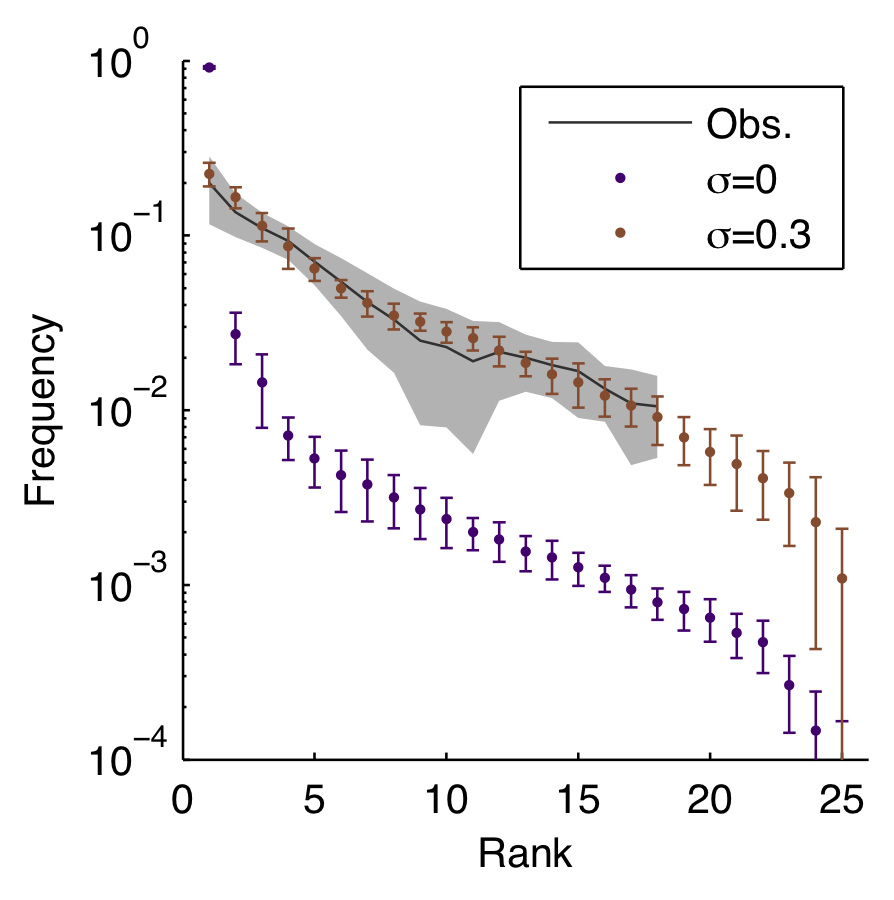### Other matched patterns

Increase in serotype diversity with age

Stable rank order

Decrease in carriage duration with age

Frequency of co-colonizations

Epidemics of rarer serotypes

### Vaccinations as natural experiments